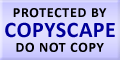Submit Assignment From Here
Name
:
Email
:
Phone
:
Country
:
Willing to pay US\$
:
Subject
:
Topic
:
Levels
:
:Hours
:

# ANOVA Homework Help

ANOVA is a statistical hypothesis testing mainly used for testing of experimental data. Basically ANOVA stands for analysis of variance; analysis of variance is nothing but a collection of many models. In these selected models, there is an observed variance which is divided into many elements and these elements are distinct from each other and they also have different source of origin. It may also be conducted to verify whether the mean of all the collected groups are equal or not. It is also useful in comparing more than one means of the groups. It generalizes t - test for more than two groups. It is helpful in making decisions related to the data analysis. Working of ANOVA is based on the designs of experiments. The experimenter collects the factors and measures required to test the functionality of the experiment. Then he checks the result from which he is able to make the correct adjustment and find an optimum effect of the factors on the experiment. ANOVA is a combination of many ideas and it has a variety of purposes. For a balanced data ANOVA will perform three processes simultaneously. ANOVA will work as an exploratory data analysis, it will side by side do the comparisons of the means of the data which is input in the ANOVA and moreover it will conduct t-tests, F-tests etc.

A diagram showing a good is provided below:US